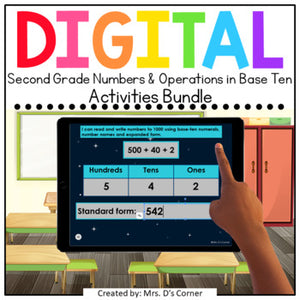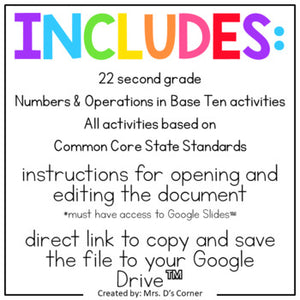FREE SHIPPING ON RUBBER STAMPS + STICKERS! BUNDLE DISCOUNT ON 2+ ITEMS!# 2nd Grade Numbers & Operations in Base Ten Digital Activity Bundle

Regular price
\$35.00
Sale price
\$35.00
Unit price
per
Shipping calculated at checkout.

SECOND GRADE NUMBERS AND OPERATIONS IN BASE TEN STANDARDS-ALIGNED DIGITAL ACTIVITIES | Functional, differentiated, and now PAPERLESS skill work that is the perfect addition to your early learning rotations and centers. This digital set of workpages will help your students practice basic skills, and once mastered, can be a set of skills they complete successfully and independently.

What is included?
• 22 (twenty-two) total digital activities (please read list below to see skills practiced)
• 220 (two hundred twenty) total digital workpages (please read list below to see skills practiced)

What skills are practiced?
• 2.NBT.A.1 - 2 activities - 20 total pages
• Level 1 - 10 pages - Build three-digit numbers using base ten blocks.
• Level 2 - 10 pages - Identify number of hundreds, tens, and ones. Circle number that blocks represent (three options).

• 2.NBT.A.2 - 2 activities - 20 total pages
• Level 1 - 10 pages - Fill in missing numbers to skip count by 5s, 10s, and 100s (ending digits of 5 or 0).
• Level 2 - 10 pages - Fill in missing numbers to skip count by 5s, 10s, and 100s.

• 2.NBT.A.3 - 2 activities - 18 total pages
• Level 1 - 6 pages - Move numbers to matching expanded form.
• Level 2 - 12 pages - Identify hundreds, tens, and ones in expanded form and write number in standard form.

• 2.NBT.A.4 - 2 activities - 20 total pages
• Level 1 - 10 pages - Move correct symbol to compare two three-digit numbers.
• Level 2 - 10 pages - Solve three-digit addition problems and move correct symbol to compare the numbers.

• 2.NBT.A.5 - 4 activities - 40 total pages
• Addition Level 1 - 10 pages - Use broken addends of two-digit numbers to solve for sum, use base ten blocks to add, solve addition sentences with regrouping.
• Subtraction level 1 - 10 pages - Use number line visual to solve subtraction problems, use base ten blocks to subtract, solve subtraction sentences with regrouping.
• Addition level 2 - 10 pages - Break addends of two-digit numbers to solve for sum, identify numbers using base ten blocks to add, solve addition sentences with regrouping.
• Subtraction level 2 - 10 pages - Use number line visual to solve subtraction problems, identify numbers using base ten blocks to subtract, solve subtraction sentences with regrouping.

• 2.NBT.B.6 - 2 activities - 20 total pages
• Level 1 - 10 pages - Add 3 and 4 two-digit numbers. Type sum.
• Level 2 - 10 pages - Add 3 and 4 two-digit numbers. Type sum.

• 2.NBT.B.7 - 4 activities - 40 total pages
• Addition Level 1 - 10 pages - Use broken addends of three-digit numbers to solve for sum, use base ten blocks to add, solve addition sentences with three-digit numbers with regrouping.
• Subtraction level 1 - 10 pages - Use base ten blocks to subtract three-digit numbers, solve subtraction sentences with three-digit numbers with regrouping.
• Addition level 2 - 10 pages - Break addends of three-digit numbers to solve for sum, identify numbers using base ten blocks to add, solve addition sentences with three-digit numbers with regrouping.
• Subtraction level 2 - 10 pages - Identify numbers using base ten blocks to subtract, solve subtraction sentences with three-digit numbers with regrouping.

• 2.NBT.B.8 - 2 activities - 22 total pages
• Level 1 - 12 pages - Type the number that is 10 more, 10 less, 100 more, and 100 less (one number per given number).
• Level 2 - 10 pages - Type 10 more and 10 less or 100 more and 100 less for each number.

• 2.NBT.B.9 - 2 activities - 20 total pages
• Level 1 - 10 pages - View strategy used to solve addition and subtraction problems. Circle if the answer is correct or incorrect.
• Level 2 - 10 pages - View strategy used to solve addition and subtraction problems. Circle mistake and rework problem to find correct answer.

Nervous or a little curious about going digital in a special needs classroom?

You can find other digital resources >> HERE <<

Connect with me:

Thanks for Looking and Happy Teaching!